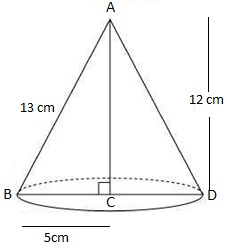# A right triangle ABC with sides 5cm, 12cm and 13cm is revolved about the side 12 cm. Find the volume of the solid so obtained.Given

Radius of cone (r) =5 cm

Height of cone (h) = 12 cm

Volume of cone = (1/3) × πr2h

= (1/3) × π × 5 × 5 × 12

= (12 × π × 5 × 5) / 3

V = 100π cm3